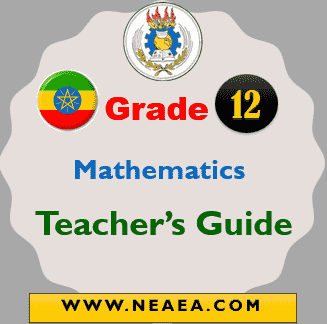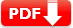# Ethiopian Grade 12 Mathematics Teacher Guide PDF

Ethiopian Grade 12 Mathematics Teacher Guide PDF: This is a free guideline book for all grade 12 Maths teachers in Ethiopia. It belongs to English Medium Schools only. The Ethiopian Grade 12 Mathematics Teacher Guide is available in PDF file bases on the new Ethiopian educational framework.

At the Grade 12 level, students should be able to: work activities with exactness and neatness with respect to the above general outcomes, the following grade-specific outcomes are expected at the end of learning Grade 12 mathematics.

## Ethiopian Grade 12 Mathematics Teacher Guide

Mathematics study at Grade 12 level is mainly aimed at exposing students to higher mathematical knowledge and competencies necessary to enable them to peruse with their higher education. The first part, which is common to both natural science and social science streams students is an introduction to calculus where the basic concepts of differential and integral calculus are introduced with intuitive explanations and examples followed by formal definitions.Units Number of Periods Unit 1: Sequences and Series 1.1 Sequences 1.2 Arithmetic sequences and geometric sequences 1.3 The sigma notation and partial sums 1.4 Infinities series 1.5 Applications of arithmetic progressions and geometric progressions Subunits 3 3 6 4 2 Total 18 Unit 2: Introduction to Limits and Continuity 2.1 Limits of sequences of numbers 2.2 Limits of functions 2.3 Continuity of a function 2.4 Exercises on applications of limits 12 6 5 3 2 28 3.1 Introduction to Derivatives   understanding rates of change  Graphical definition of the derivative  Formal definition (Differentiability at a point)  Differentiability over an interval 3.2 Derivatives of different functions.  Differentiation of power, simple trigonometric, exponential and logarithmic functions. 3.3 Derivatives of combinations and compositions of functions 3.4 Miscellaneous exercise 10   3   12   2 27 Unit 4 Applications of Differential Calculus 4.1 Extreme values of functions 4.2 Minimization and maximization problems 4.3 Rate of change 13 6 6 25 Unit 5: Introduction to Integral Calculus 5.1 Integration as inverse process of differentiation  Integral of: – Constant – Power – Trigonometric – Exponential and logarithmic functions 5.2 Techniques of integration  Elementary substitution  Partial fractions  Integration by parts 5.3 Definite integrals, area and fundamental theorem of calculus 7     9   8 30 Unit 6: Three Dimensional Geometry and Vectors in Space (For Natural Science Students) 6.1 Coordinate axes and coordinate planes in space 6.2 Coordinates of a point in space 6.3 Distance between two points in space 6.4 Mid-point of a line segment in space 6.5 Equation of sphere 6.6 Vectors in space 2 2 2 1 2 8 17 Unit 7: Mathematical Proofs (For Natural Science Students) 7.1 Revision on logic 7.2 Different types of proofs 7.3 Principle and application of mathematical induction 5 4 4 2 15 Unit 8 Further on Statistics (For Social Science Students) 8.1 Sampling techniques 8.2 Representation of data 8.3 Construction of graphs and interpretation 8.4 Measures of central tendency and variation of a set of data, including grouped data. (Mean, Median, Mode, Range, Inter quartile rang and Standard deviation from the data itself or from given totals) 8.5 Analysis of frequency distributions with equal means but different variances (coefficients of variation). 8.6 Use of cumulative frequency graph to estimate 3 2 6 5   2   4 22 Unit 9 Mathematical Applications for Business and consumers (For Social Science Students)  9.1 Applications to purchasing 9.2 Percent increase and percent decrease 9.3 Real estate expenses 9.4 Wages 3 4 4 4 15

 Country Ethiopia Publisher Curriculum Framework Development Department of the MOE Grade Grade 12 Subject Maths Teacher Guide Type Teacher’s Guide Purpose Teaching & Learning Download Link